# How to use the WEIBULL.DIST function in Excel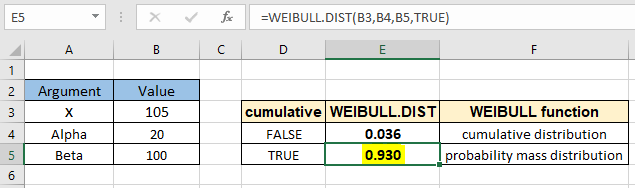In this article, we will learn How to use the WEIBULL.DIST function in Excel.

What is Weibull distribution?

The Weibull distribution is a two-parameter family of continuous probability distributions. It is named after Swedish mathematician Waloddi Weibull. This type of distribution is generally used for the distribution in reliability analysis or the analysis of time to failure. For example finding the mean time of medical and dental implants or Components produced in a factory (like bearings, capacitors, or dielectrics) or warranty analysis for a product. The function depends on the two parameters lambda and k. Now let's see how the parameters affect the probability factor. Gamma distribution(CDF) can be carried out in two types one is cumulative distribution function, the mathematical representation and weibull plot is given below.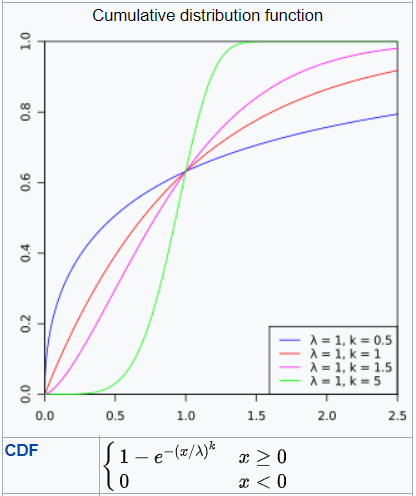You can see the effect of changing parameters with different color lines as indicated in the plot with the mathematical formula stated below it.

Now we proceed to the other part which is probability density function which is extracted when you differentiate the above equation. We get the probability mass distribution plot and mathematical formula stated below.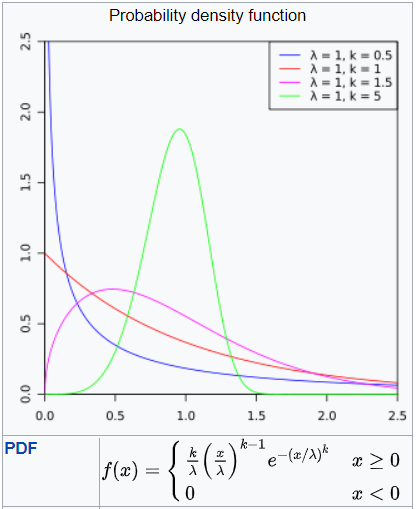when plotted on graph having given parameters lambda  and k.

The gamma distribution plot is shown above. As you can see these are complex functions to extract probability using it in excel. So excel provides the inbuilt statistical function to get weibull distribution function results. Let's understand how to use the WEIBULL.DIST function to work as an weibull distribution calculator.

WEIBULL.DIST Function in Excel

WEIBULL.DIST is a statistical function which returns the weibull distribution at a particular value. It takes the value and the two parameters named alpha and beta along with the type of distribution required(cdf or pdf). Let's understand these arguments with the function syntax as explained below.

WEIBULL.DIST Function syntax :

 =WEIBULL.DIST(x, alpha, beta, cumulative)

x : value at which you want to evaluate the distribution.

alpha : parameter for the weibull distribution. If alpha = 1, WEIBULL.DIST acts as the exponential distribution function.

beta : parameter for the weibull distribution.

cumulative : logical value that determines the form of the function. If cumulative is TRUE, WEIBULL.DIST returns the cumulative distribution function; if FALSE, it returns the probability density function.

Example :

All of these might be confusing to understand. Let's understand how to use the function using an example. Here we have the x value along with alpha and beta parameters for the weibull distribution stated below.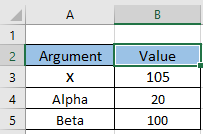Now using these parameters, we will evaluate the cumulative distribution for the weibull function with the formula stated below.

Use the formula:

 =WEIBULL.DIST(B3,B4,B5,FALSE)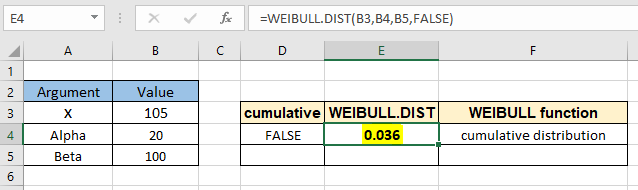As you can see, the formula returns the cumulative probability value exactly at 105 comes out to be 0.036 or 3.6%.

Now to calculate the value for at least 105 we can use the probability mass distribution formula by changing the cumulative argument. Use the formula to find the probability for weibull distribution given the same parameters.

Use the formula:

 =WEIBULL.DIST(B3,B4,B5,TRUE)As you can see, the formula returns the cumulative probability value  for at least 105 comes out to be 0.930 or 93%.

Here are all the observational notes using the WEIBULL.DIST function in Excel
Notes :

1. The function only works with numbers. If any argument other than cumulative is non numeric, the function returns #VALUE! error.
2. The function returns #NUM! Error.
1. If x < 0
2. If alpha < =0
3. If beta < = 0
3. The cumulative argument can be used with boolean numbers (0 and 1) or (FALSE or TRUE).
4. You can feed the arguments to the function directly or using the cell reference as explained in the example.

Hope this article about How to use the WEIBULL.DIST function in Excel is explanatory. Find more articles on statistical formulas and related Excel functions here. If you liked our blogs, share it with your friends on Facebook. And also you can follow us on Twitter and Facebook. We would love to hear from you, do let us know how we can improve, complement or innovate our work and make it better for you. Write to us at info@exceltip.com.

Related Articles :

How to use Excel NORM.DIST Function : Calculate the Z score for the normal cumulative distribution for the pre specified values using the NORMDIST function in Excel.

How to use Excel NORM.INV Function : Calculate the inverse of Z score for the normal cumulative distribution for the pre-specified probability values using the NORM.INV function in Excel.

How to Calculate Standard Deviation in Excel : To calculate the standard deviation we have different functions in Excel. The standard deviation is the square root of the variance value but It tells more about the dataset than variance.

Regressions Analysis in Excel : Regression is an Analysis Tool, which we use for analyzing large amounts of data and making forecasts and predictions in Microsoft Excel.

How to Create Standard Deviation Graph : The standard deviation tells how much data is clustered around the average of the data. Learn how to create a standard deviation graph here.

How to use the VAR function in Excel : Calculate the variance for the sample dataset in excel using the VAR function in Excel.

Popular Articles :

How to use the IF Function in Excel : The IF statement in Excel checks the condition and returns a specific value if the condition is TRUE or returns another specific value if FALSE.

How to use the VLOOKUP Function in Excel : This is one of the most used and popular functions of excel that is used to lookup value from different ranges and sheets.

How to use the SUMIF Function in Excel : This is another dashboard essential function. This helps you sum up values on specific conditions.

How to use the COUNTIF Function in Excel : Count values with conditions using this amazing function. You don't need to filter your data to count specific values. Countif function is essential to prepare your dashboard.

Terms and Conditions of use

The applications/code on this site are distributed as is and without warranties or liability. In no event shall the owner of the copyrights, or the authors of the applications/code be liable for any loss of profit, any problems or any damage resulting from the use or evaluation of the applications/code.